Берзин Д.В.

Кандидат физико-математических наук, доцент, Финансовый университет при Правительстве Российской Федерации, Москва

О ВАРИАЦИОННОЙ ЗАДАЧЕ ДЛЯ NURBS ПОВЕРХНОСТИ

Аннотация

Предположим, что мы хотим изменить (деформировать) NURBS минимальным образом, чтобы достичь условия непрерывности с ее соседями. В данной работе формулируется проблема соблюдения условия непрерывности G и выписываются соответствующие уравнения.

Ключевые слова: система автоматизированного проектирования, условие непрерывности G,  NURBS, вариационная задача.

Berzin D.V.

PhD, Associate Professor, Financial University under the Government of the Russian Federation, Moscow

ABOUT A VARIATIONAL PROBLEM FOR NURBS SURFACE

Abstract

Suppose we want to deform a base surface of a face in order to achieve some continuity condition (e.g., G continuity) with the given neighbors at common edges. We formulate a problem for preserving G continuity and present equations for a deformation that changes the surface geometry as little as possible.

Keywords: CAD, G continuity, NURBS, variational problem.

Suppose that a face F0 is surrounded by some number of neighbor faces F1, F2, … . We want to deform an (initial) base surface of F0 in order to achieve some continuity condition (e.g., G continuity) with the given neighbors at common edges. This deformation should change the surface geometry as little as possible.

1. “Curve error” functional

Denote vectors of initial and deformed control points by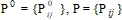respectively. Consider a curve c0(t), which belongs to (or located near) the initial (not deformed) surface S(P0). Let

w = w(c0, t) = (u(t),(v(t))                (1)

be a corresponding uv-curve of c0(t). Consider a class of 3D-curves with a fixed w and the variable P: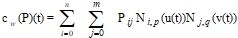(2)

Consider a functional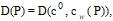(3)

which in some way expresses distance (or maximum gap) between initial and deformed curves. Let call such a functional “curve error” functional.

2. Other functionals

Consider two more types of functionals: H(P) and G(P). “Control point error” functional H(P) expresses a distance between sets of control points P0 and P. H(P) is to control a deviation of a deformed surface. “Continuity error” functional G(P) is to keep some continuity condition, for example, G1 with some of neighbor faces.

3. Quasi-G

Instead of G1 at sample points on boundary curves, we can try to achieve a little different (and, in some meaning, stronger, than G1) condition, which, however, leads to linearity in the variational problem. Let E be an arbitrary, but fixed sample point on some edge, which is shared by both face F0 and the neighbor face F1. Consider a tangent plane π at E to a base surface of the face F1. Let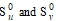be corresponding tangent vectors (taken at the point E in u and v directions respectively) to the initial base surface S(P0) of the F0. Projectonto π, get the pair of vectors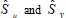respectively. Now we can compose the continuity error functional for this condition at the point E:

G(P) = || S – || + || S -||,               (4)

where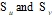– corresponding tangent vectors to the deformed surface S(P), and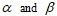are real variables. Respectively, continuity error functional for a set of sample points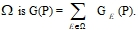4. Variational problem

Now, we can compose the “total error” functional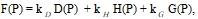(5)

where constants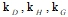can serve as weights and might be found empirically. Eventually, our goal is to find a minimum: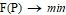(6)

This variational problem without restrictions (see ) can be solved according to the Fermat theorem:(7)

where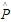is a solution of the problem.

5. Remarks

In this approach, knot vectors and the number of control points are still the same after deformation. Perhaps, this restriction will not allow achieving a precise continuity condition and preserving boundary curves within prescribed tolerances. It is needed to measure continuity and curve errors, and, if necessary, insert additional knots in the initial surface, and after that restart the deformation.

All terms in (5) should have a quadratic form, so that the system (7) becomes linear. In our first implementation, we will assume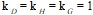for simplicity.

Quasi-G1 condition is not the same as G1, but we can expect that generally (6) will force the corresponding tangent planes to approach desired positions.  In  we gave an algorithm for such a deformation.

References

1. W. Welch, A. Witkin “Variational Surface Modeling” // Computer Graphics　(ACM), 1992
2. G. Celniker, W. Welch “Linear constraints for deformable B-spline surfaces” // Computer Graphics, 1992
3. D. Terzopoulos, H. Qin “Dynamic NURBS with geometric constraints for interactive sculpting” // ACM Transactions on Graphics, 1994
4. S. V. Fomin, I. M. Gelfand “Calculus of Variations” // Dover Publications, 2000
5. Berzin D.V. “Surface deformation with geometric constraints” // Research Journal of International Studies, №8 (15) 2013, part 1, p.6